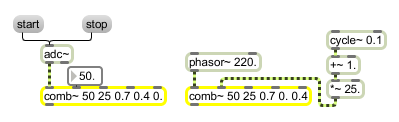# comb~

Comb filter

## Description

comb~ mixes the current input sample with earlier input and/or output samples, according to the formula:

yn = axn + bxn-(DR/1000) + cyn-(DR/1000)

where R is the sampling rate and D is a delay time in milliseconds.

## Arguments

Name Type Opt Description
filter-parameters (up to 5 floats) list opt Up to five numbers, to set the maximum delay time and initial values for the delay time D and coefficients a, b, and c. If a signal is connected to a given inlet, the coefficient supplied as an argument for that inlet is ignored. If no arguments are present, the maximum delay time defaults to 10 milliseconds, and all other values default to 0.

## Messages

 int filter-parameters [int] The filter parameters in inlets 2 to 5 may be specified by a int instead of a signal. If a signal is also connected to the inlet, the int is ignored. float filter-parameters [float] The filter parameters in inlets 2 to 5 may be specified by a float instead of a signal. If a signal is also connected to the inlet, the float is ignored. clear Clears the comb~ object's memory of previous outputs, resetting them to 0. signal In left inlet: Signal to be filtered. In 2nd inlet: Delay time (D) in milliseconds for a past sample to be added into the current output. In 3rd inlet: Amplitude coefficient (a), for scaling the amount of the input sample to be sent to the output. In 4th inlet: Amplitude coefficient (b), for scaling the amount of the delayed past input sample to be added to the output. In right inlet: Amplitude coefficient (c), for scaling the amount of the delayed past output sample to be added to the output. list filter-parameters [list] The three parameters can be provided as a list in the left inlet. The first number in the list is the delay time D, the next number is coefficient a, and the third number is coefficient b. If a signal is connected to a given inlet, the coefficient supplied in the list for that inlet is ignored.

## Output

signal: The filtered signal.

## ExamplesFilter parameters may be supplied as float values or as signals